# How to use the wildcards in Excel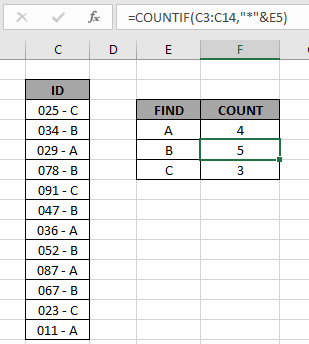In this article, we will learn How to use the wildcards in Excel.

Partial match up in Excel

In Excel, sometimes you find yourself with problems where you need to perform an operation over some values having criteria. For example finding the sum of sales of employees who belong from the B department where department names are included in Employee ID (0234-B-2019). For these kinds of problems, excel provides you with three wildcards.

1. Question mark ( ? ) : This wildcard is used to search for any single character. This is used when you know the exact count of matchup value. Like matching phone numbers (8 digit) which starts with 999 (use "999?????".
2. Asterisk ( * ): This wildcard is used to find any number of characters preceding or following any character. This is the most used wildcard. Like matching domain names in urls (use "*@domain.*) .
3. Tilde ( ~ ): This wildcard is an escape character, used preceding the question mark (?) or asterisk mark (*). This used when we need to match * or ? chars.

These can be used either in criterias or in the filter options to filter directly using wildcard. Let's understand how to use them with functions or with filter tool to filter results.

Example :

All of these might be confusing to understand. Let's understand how to use the function using an example. Here we have the ID records and we need to find the IDs which ends with A category.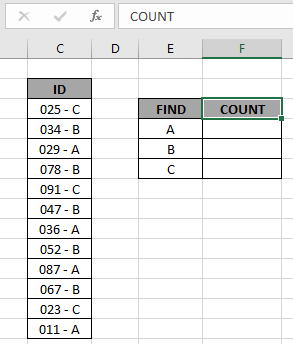Here to find the A category IDs, will be using the * (asterisk) wildcard in the formula. * (asterisk) wildcard finds any number of characters within lookup value.

Use the formula:

 = COUNTIF ( C3:C14, "*A" )

OR

 = COUNTIF ( C3:C14, "*" & E4 )

Explanation:

• COUNTIF function count the cells given criteria
• Criteria is given in using * (asterisk) wildcard to look for value which has any number of characters.
• & operator concatenate the two values where it lays
• "*" & E4 : criteria, formula checks each cell and returns only the cells which satisfies the criteria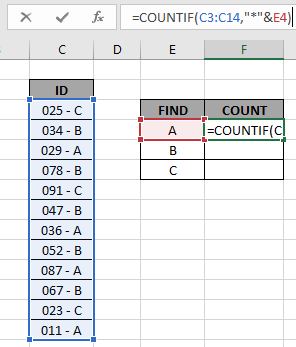Here the range is given as array reference and pattern is given as cell reference. Press Enter to get the count.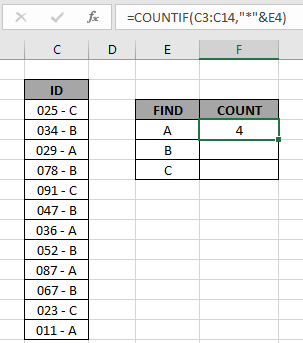As you can see the total values which ends with value A comes out to be 4.

Now copy the formula in other cells using the drag down option or using the shortcut key Ctrl + D as shown below.As you can see all the different category count of IDs is there.

You can check which values end with a specific pattern in range using the excel filter option. Apply the filter to the ID header and click the arrow button which appears. Follow the steps as shown below.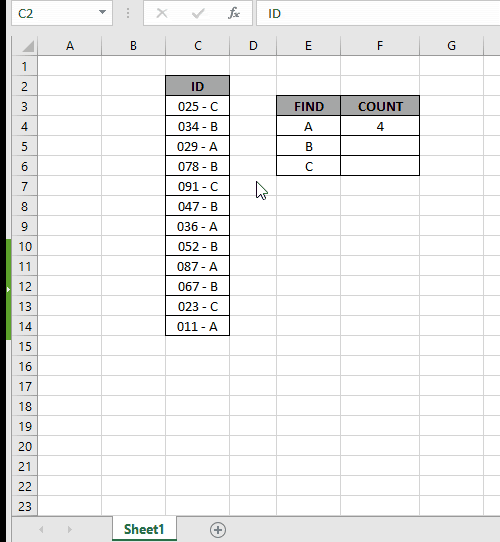Steps:

1. Select the ID header cell. Apply filter using shortcut Ctrl + Shift + L
2. Click the arrow which appeared as a filter option.
3. Deselect (Select All) option.
4. Now search in search box using the * (asterisk) wildcard.
5. Type *A in the search box and select the required values or select the (Select All search result) option as shown in the above gif.

IF function with wildcards

Here we have company codes on one side. And we need to find the fields where the phrase AT is present anywhere in the code.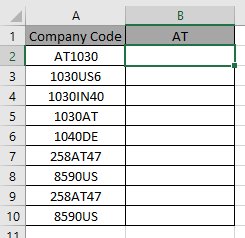IF function doesn’t support wildcards so we will be using SEARCH function. SEARCH function returns a number if the phrase is present within the text.

Use the formula:

 =IF(ISNUMBER(SEARCH("*AT*",A2)), "AT", "")

SEARCH function accepts the wildcard (*) and finds the phrase “AT”, within A2. It returns a number if SEARCH finds the phrase.

ISNUMBER function finds the number and returns TRUE.

IF function logic_test results in TRUE and FALSE and returns “AT” if True or “”(empty string) if False.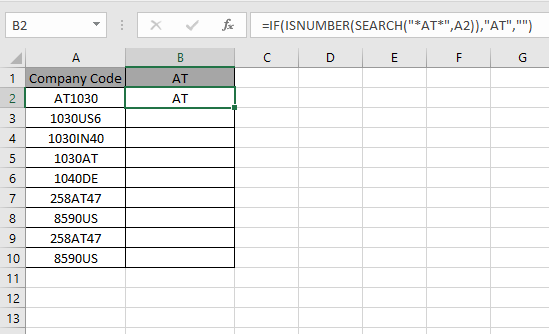As you can the formula catches the AT in A2 cell and returns the phrase. This shows A2 cell has “AT” pharse within text.

Now use the formula in other cells to get all fields containing the phrase “AT”.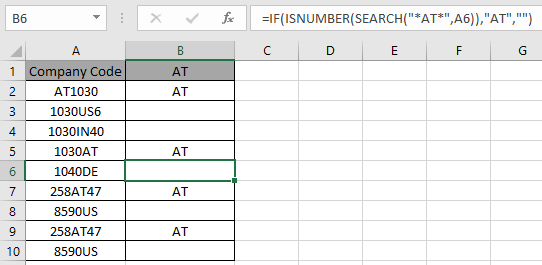As you can see we used wildcards with IF function to get the result.

Hope this article about How to use the wildcards in Excel is explanatory. Find more articles on calculating values and related Excel formulas here. If you liked our blogs, share it with your friends on Facebook. And also you can follow us on Twitter and Facebook. We would love to hear from you, do let us know how we can improve, complement or innovate our work and make it better for you. Write to us at info@exceltip.com.

Related Articles :

Replace text from end of a string starting from variable position: To replace text from the end of the string, we use the REPLACE function. The REPLACE function uses the position of text in the string to replace.

How to Check if a cell contains one of many texts in Excel: To find check if a string contains any of multiple text, we use this formula. We use the SUM function to sum up all the matches and then perform a logic to check if the string contains any of the multiple strings.

Count Cells that contain specific text: A simple COUNTIF function will do the magic. To count the number of multiple cells that contain a given string we use the wildcard operator with the COUNTIF function.

Excel REPLACE vs SUBSTITUTE function: The REPLACE and SUBSTITUTE functions are the most misunderstood functions. To find and replace a given text we use the SUBSTITUTE function. Where REPLACE is used to replace a number of characters in string.

How to use wildcards in excel : Count cells matching phrases using the wildcards in excel

Popular Articles :

How to use the IF Function in Excel : The IF statement in Excel checks the condition and returns a specific value if the condition is TRUE or returns another specific value if FALSE.

How to use the VLOOKUP Function in Excel : This is one of the most used and popular functions of excel that is used to lookup value from different ranges and sheets.

How to use the SUMIF Function in Excel : This is another dashboard essential function. This helps you sum up values on specific conditions.

How to use the COUNTIF Function in Excel : Count values with conditions using this amazing function. You don't need to filter your data to count specific values. Countif function is essential to prepare your dashboard.

Terms and Conditions of use

The applications/code on this site are distributed as is and without warranties or liability. In no event shall the owner of the copyrights, or the authors of the applications/code be liable for any loss of profit, any problems or any damage resulting from the use or evaluation of the applications/code.